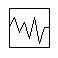# Controller -- Noise

Navigation: Main Page -> Models -> Control 2 Models

Related Links: Noise Class

NOTE this page is only valid for SysCAD 9.3 or later versions. For Help for SysCAD 9.2, see Controller -- Noise (SysCAD 9.2)

## General Description

• This unit operation is used to simulate process noise. It can be used to add disturbance to Feeders, pipe outlet capacity and other writeable variables.
• The Noise Control model is an orphan model, i.e it cannot be connected to any other unit operation.
• The noise model is for dynamic simulation only with the purpose of adding disturbance to the model.

### DiagramThe diagram shows the default drawing of the Noise control unit.

## Inputs and Outputs

There are no connections to this unit.

## Model Theory

In SysCAD 9.3 Build 136 or earlier, the random number generator used for these probability functions is from Numerical Recipes in C.

In SysCAD 9.3 Build 137 or later, the random number generator used for all probability functions is from the C++ standard library (http://www.cplusplus.com/reference/random/).

The Probability Density functions used for the Noise generation are shown in the following headings. In all cases the following convention is used:

$\mu$ = Mean Value
$\sigma$ = Standard Deviation

### Gaussian

The Probability Density Function P(t):

$P(t) = \frac{1}{\sigma \sqrt{2π}}e^{\dfrac{-(t-\mu)^2}{2\sigma^2}}$
where $\sigma \gt 0$

### Flat

The Probability Density Function P(t):

$P(t) = 0$
where $t \lt \mu - m$ or $t \gt \mu + m$
$P(t) = \frac{1}{2\sigma}$
where $\mu - m ≤ t ≤ \mu + m$ and $m ≥ 0$
and $m$ = maximum deviation

### Poisson

The Probability Density Function P(t):

$P(t) = \frac{\mu^t}{t!}\ e^{-\mu}$
where $e$ = the base of the natural logarithm system (2.71828...) and $\mu \gt 0$

### Gamma

The Probability Density Function (P(t)):

$P(t) = \frac{1}{\Gamma(\alpha)\ \beta^{\alpha}}\ t^{\alpha-1}\ e^{-t/\beta}$
where $\alpha ≥ 0.5$ and $\beta \gt 0$

Note: The Chi-squared distribution is a special case of the Gamma distribution with $\alpha = n/2$ and $\beta = 2$, where $n$ = degrees of freedom

### Weibull

The Probability Density Function (P(t)):

$P(t) = \alpha^{-\beta}\ \beta\ t^{\beta-1}\ e^{-(t/\alpha)^\beta}$
where $t = \alpha (-\ln(U))^{1/\beta}$ and $\alpha ≥ 0.04$ and $\beta ≥ 0.01$
$U$ is a randomly generated number between 0 and 1

### Bernoulli

Only available in SysCAD 9.3 Build 137 or later.

The Probability Density Function (P(t)):

$P(t) = p^{t}\ (1-p)^{1-t}$
where $0 ≤ p ≤ 1$

### Binomial

Only available in SysCAD 9.3 Build 137 or later.

The Probability Density Function (P(t)):

$P(t) = \binom{n}{t} p^{t}\ (1-p)^{n-t}$
where $n$ is a positive integer, $\binom{n}{t} = \frac{n!}{t!(n-t)!}$ and $0 \lt p ≤ 1$

### Geometric

Only available in SysCAD 9.3 Build 137 or later.

The Probability Density Function (P(t)):

$P(t) = p\ (1-p)^{t}$
where $0 \lt p ≤ 1$

### Exponential

Only available in SysCAD 9.3 Build 137 or later.

The Probability Density Function (P(t)):

$P(t) = \mu\ e^{-\mu\ t}$
where $\mu \gt 0$

### Extreme Value

Only available in SysCAD 9.3 Build 137 or later.

The Probability Density Function (P(t)):

$P(t) = \frac{1}{\beta}\ z(t)\ e^{-z(t)}$
where $z(t) = e^{(\alpha-t)/\beta}$ and $\beta \gt 0$

### Log Normal

Only available in SysCAD 9.3 Build 137 or later.

The Probability Density Function (P(t)):

$P(t) = \frac{1}{s\ t\ \sqrt{2π}}\ e^{-(\ln t-m)^2/2s^2}$
where $0 \lt s ≤ 50.70$

### Cauchy

Only available in SysCAD 9.3 Build 137 or later.

The Probability Density Function (P(t)):

$P(t) = \frac{1}{π\ \beta\ \left [1+\left (\frac{t-\alpha}{\beta} \right )^2 \right ]}$
where $\beta \gt 0$

### Fisher F

Only available in SysCAD 9.3 Build 137 or later.

The Probability Density Function (P(t)):

$P(t) = \frac{Γ \left (\frac{m+n}{2} \right )}{Γ\left (\frac{m}{2}\right )\ Γ\left (\frac{n}{2}\right )}\ \frac{\left (\frac{m\ t}{n} \right )^{(m/2)}}{t\ \left (1+\frac{m\ t}{n} \right )^{(m+n)/2}}$
where $1 ≤ m ≤ 100$ and $n ≥ 1$

### Student t

Only available in SysCAD 9.3 Build 137 or later.

The Probability Density Function (P(t)):

$P(t) = \frac{1}{\sqrt{n\ π}}\ \frac{Γ\left (\frac{n+1}{2} \right )}{Γ\left (\frac{n}{2} \right )}\ \left (1+\frac{t^2}{n} \right )^{-(n+1)/2}$
where $n ≥ 0.25$